# ZYMIN

0%

## 数值点之间的距离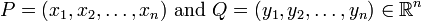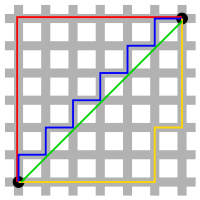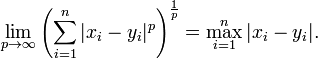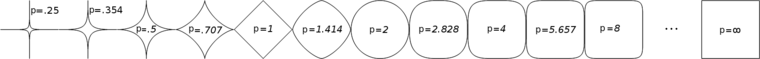$(x_1,y_1)\mapsto(\frac{x_1-\mu_x}{\sigma_x},\frac{y_1-\mu_y}{\sigma_y})$

$$\mu$$ : 该维度上的均值 $$\sigma$$: 该维度上的标准差

### 欧氏距离(Euclidean Distance)

#### (1)二维平面上两点a$$(x_1,y_1)$$与b$$(x_2,y_2)$$间的欧氏距离：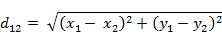#### (2)两个n维向量a$$(x_11,x_12,…,x_1n)$$与 b$$(x_21,x_22,…,x_2n)$$间的欧氏距离：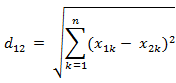#### (3)也能够用表示成向量运算的形式：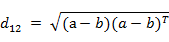#### (4)Matlab计算欧氏距离

Matlab计算距离主要使用pdist函数。 若X是一个M×N的矩阵。则pdist(X)将X矩阵M行的每一行作为一个N维向量。然后计算这M个向量两两间的距离。 样例：计算向量(0,0)、(1,0)、(0,2)两两间的欧式距离

### 曼哈顿距离(Manhattan Distance)

#### (1)二维平面两点a:$$(x_1,y_1)$$与b:$$(x_2,y_2)$$间的曼哈顿距离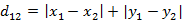#### (2)两个n维向量a$$(x_{11},x_{12},…,x_{1n})$$与 b$$(x_{21},x_{22},…,x_{2n})$$间的曼哈顿距离## 切比雪夫距离 ( Chebyshev Distance )

#### (1)二维平面两点a$$(x_1,y_1)$$与b$$(x_2,y_2)$$间的切比雪夫距离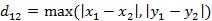#### (2)两个n维向量a$$(x_{11},x_{12},…,x_{1n})$$与 b$$(x_{21},x_{22},…,x_{2n})$$间的切比雪夫距离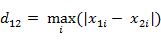这个公式的还有一种等价形式是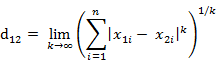## 闵可夫斯基距离(Minkowski Distance)

#### (1) 闵氏距离的定义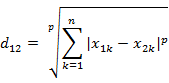####（1）马氏距离定义

## 夹角余弦(Cosine)

#### (1)在二维空间中向量a$$(x_1,y_1)$$与向量b$$(x_2,y_2)$$的夹角余弦公式：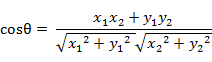#### (2) 两个n维样本点a(x_11,x_12,…,x_1n)和b(x_21,x_22,…,x_2n)的夹角余弦：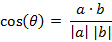类似的，对于两个n维样本点a(x_11,x_12,…,x_1n)和b(x_21,x_22,…,x_2n)，能够使用类似于夹角余弦的概念来衡量它们间的类似程度。 　　即：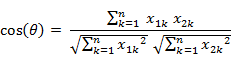夹角余弦取值范围为[−1,1]。夹角余弦越大表示两个向量的夹角越小，夹角余弦越小表示两向量的夹角越大。 当两个向量的方向重合时夹角余弦取最大值1，当两个向量的方向全然相反夹角余弦取最小值-1。

## 分类数据点之间的距离

### 汉明距离(Hamming distance)

#### (2)Matlab计算汉明距离

Matlab中2个向量之间的汉明距离的定义为2个向量不同的分量所占的百分比。 样例：计算向量(0,0,0)、(0,0,1)、(0,1,0)、(1,0,0)、(0,1,1)两两间的汉明距离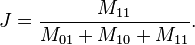Jaccard similarity 还可以用集合的公式来表达。

## 杰卡德类似系数(Jaccard similarity coefficient)

#### (4)Matlab 计算杰卡德距离

Matlab的pdist函数定义的杰卡德距离跟我这里的定义有一些区别，Matlab中将其定义为不同的维度的个数占“非全零维度”的比例。 样例：计算(1,1,0)、(1,−1,0)、(−1,1,0)两两之间的杰卡德距离

### 序列之间的距离(字符串、时序)

DTW 距离在保持信号先后顺序的限制下对时间信号进行“膨胀”或者“收缩”，找到最优的匹配，与编辑距离相似，这其实也是一个动态规划的问题:

## 相关系数 ( Correlation coefficient )与相关距离(Correlation distance)

#### (1) 相关系数的定义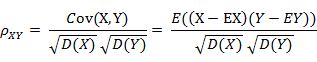相关系数是衡量随机变量X与Y相关程度的一种方法，相关系数的取值范围是[−1,1]。相关系数的绝对值越大，则表明X与Y相关度越高。 当X与Y线性相关时，相关系数取值为1（正线性相关）或-1（负线性相关）。

#### (2)相关距离的定义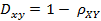## 混合分类数据点与数字数据点之间的距离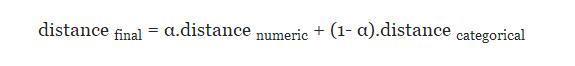## 概率分布之间的距离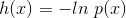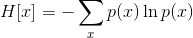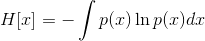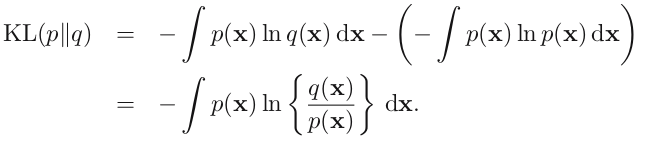KL 散度又叫相对熵（relative entropy）。了解机器学习的童鞋应该都知道，在 Softmax 回归（或者 Logistic 回归），最后的输出节点上的值表示这个样本分到该类的概率，这就是一个概率分布。对于一个带有标签的样本，我们期望的概率分布是：分到标签类的概率是 1， 其他类概率是 0。但是理想很丰满，现实很骨感，我们不可能得到完美的概率输出，能做的就是尽量减小总样本的 KL 散度之和（目标函数）。这就是 Softmax 回归或者 Logistic 回归中 Cost function 的优化过程啦。（PS：因为概率和为 1，一般的 logistic 二分类的图只画了一个输出节点，隐藏了另外一个）

## 向量内积

$Inner(x,y)=\langle x,y\rangle=\sum_ix_iy_i$

$CosSim(x,y)=\frac{\sum_ix_iy_i}{\sqrt{\sum_ix_i^2}\sqrt{\sum_iy_i^2}}=\frac{\langle x,y\rangle}{||x||\||y||}$

\begin{align}Corr(x,y)&=\frac{\sum_i(x_i-\bar{x})(y_i-\bar{y})}{\sqrt{\sum(x_i-\bar{x})^2}\sqrt{\sum(y_i-\bar{y})^2}}\\&=\frac{\langle x-\bar{x},y-\bar{y}\rangle}{||x-\bar{x}||\||y-\bar{y}||}\\&=CosSim(x-\bar{x},y-\bar{y})\end{align}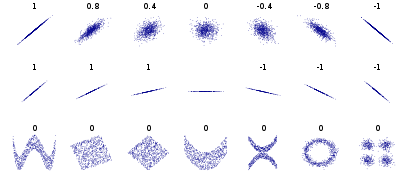Machine Learning: Measuring Similarity and Distance

Computing and Visualizing Dynamic Time Warping Alignments in R: The dtw Package